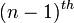# N-sphere is (n-1)-connected

Jump to: navigation, search

## Statement

Suppose$n$ is a natural number (i.e.,$n \ge 1$). Then, the$n$-Sphere (?) is$(n - 1)$-connected. In other words:

• For$n = 1$, the$n$-sphere, better known as the circle, is a path-connected space.
• For$n \ge 2$, the$n$-sphere is a Path-connected space (?) and Simply connected space (?) and all its homotopy groups up to the$(n - 1)^{th}$ homotopy group are trivial. In other words,$\pi_1(S^n), \pi_2(S^n), \dots, \pi_{n-1}(S^n)$ are all trivial.

By the Hurewicz theorem, this is equivalent (for$n \ge 2$) to the assertion that$S^n$ is simply connected and the first$n - 1$ homology groups are trivial.